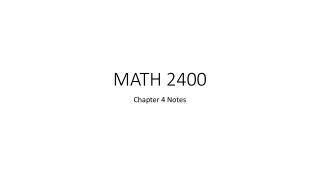Download PresentationMATH 2400

# MATH 2400 - PowerPoint PPT PresentationDownload Presentation## MATH 2400

- - - - - - - - - - - - - - - - - - - - - - - - - - - E N D - - - - - - - - - - - - - - - - - - - - - - - - - - -
##### Presentation Transcript

1. MATH 2400 Chapter 4 Notes

2. Response & Explanatory Variables A response variable (a.k.a. dependent variables) measures an outcome of a study. An explanatory variable (a.k.a. independent variables or predictor variables) may explain or influence changes in a response variable. Ex: Student volunteers drank different numbers of cans of beer. Thirty minutes later, a police officer measured their blood alcohol content (BAC). What is the response variable and the explanatory variable?

3. Example 1 A college student aid officer looks at some data. She looks at data regarding the amount of debt of recent graduates, their current income, and how stressed they feel about the debt. She isn’t interested in predictions but is simply trying to understand the situation of recent college graduates. The distinction between explanatory and response variables does not apply. A sociologist looks at the same data with an eye to using amount of debt and income, along with other variables, to explain the stress caused by college debt. Now, amount of debt and income are explanatory variables and stress level is the response variable.

4. Scatterplots Used to show the relationship between two quantitative variables measured on the same individuals. The explanatory variable, if there is one, is always plotted on the horizontal axis. Ex: The following is data from 12 women who are subjects in a study of dieting to study how the body consumes energy. Lean body mass, given in kilograms, is a person’s weight leaving out all fat. Metabolic rate is measured in calories burned per 24 hours. Researchers believe that lean body mass is an important influence on the metabolic rate. Make a scatterplot to examine this belief.

5. What Scatterplots Tell Us Important things to notice: • Direction (positive or negative) • Form (linear, exponential, quadratic, etc.) • Strength (how well the relationship “fits”)

6. Example 2 Many manatees are injured or killed by boats along the coast of Florida. The following data represents the number of boats registered in Florida (in thousands) and the number of manatees killed by boats for the years between 1977 and 2009. Is it plausible that restricting the number of boats would help protect manatees?

7. Example 2 continued… In this scatterplot, there is a positivelinear relationship because the pattern follows a straight line from the lower left to the upper right. The relationship is strong because the points don’t deviate greatly from the line.

8. Example 3 The study mentioned on slide 4 collected data for both male and female subjects. a) Make a scatterplot of metabolic rate versus lean body mass for all 19 subjects. Use separate symbols to distinguish men and women. b) Does the same overall pattern hold for both men and women?

9. Correlation Correlation describes how well data fits a model. To formula to calculate the correlation coefficient is… If r is positive, there exists a positive correlation between the response variable and the explanatory variable (y and x). Negative r implies a negative correlation. The closer r is to 1 or -1, the stronger the correlation. The closer ris to 0, the weaker the correlation.

10. Values for r |r| ≥ .70  Very Strong relationship .40 ≤ |r| ≤ .69  Moderate relationship .20 ≤ |r| ≤ .29  Weak relationship .01 ≤ |r| ≤ .19  No relationship

11. Example 4 The following is the mean coral growth in millimeters per year over a several-year period at locations in the Red Sea. • Make a scatterplot. Which is the explanatory variable? Is there a negative or positive correlation? • Find the correlation coefficient r.

12. Facts About Correlation • No distinction between explanatory (x) and response (y) variables. • r has no unit. Also, it doesn’t matter what units x & y are in (cm, feet, inches, etc.), r would be calculated to be the same value either way. • Positive r  positive correlation Negative r  negative correlation • Always a number between -1 and 1. Values near 0 have a very weak linear relationship. The strength of the relationship increases as r moves away from 0 towards either -1 or 1. Values for r = -1 and r = 1 only occur for perfect linear relationships. Take a look in the book at Example 4.6 for various examples associated with various values for r.